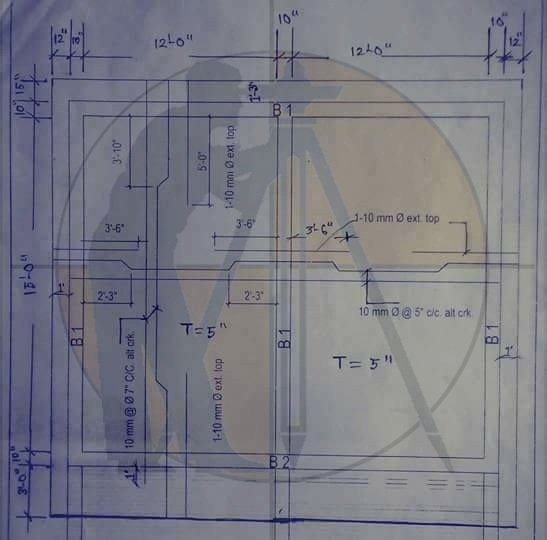Breaking News
Home / Civil Engineering / Bar Bending Schedule / Estimate this Slab Included Drawing

# Estimate this Slab (Drawing Included).

Questions:- Estimate this Slab (Drawing Included).Calculation:-
Estimate for Slab Casting (1:2:4).
= 1×28′-6″x20′-11″x0′-5″ = 247.98 Cft. = 7.021 Cum.
Estimate for Formwork.
Soffit = 1×28′-6″x20’11” = 596.10 Sft.
Side = 1×2(28′-6″+20′-11″)x0′-5″ = 41.11 Sft.
Total Formwork = 637.21 Sft. = 59.22 Sqm.

Estimate for Reinforcement.
Short Direction Rod:-
10 mm @ 5″ C/C Alt Ckd.
Ckd = 1x19x29′-0″ = 551.00 Rft.
St = 1x18x26′-9″ = 481.50 Rft
Ext Top = 2x18x5′-4″ = 191.98 Rft.
= 1x18x7′-10″ = 141.00 Rft.
Binder = 15×28′-6″ = 427.50 Rft.

## Other Post

3. ### How do you take off a Staircase

Long Direction Rod:-
10mm @ 7″ C/C Alt Ckd.
Ckd = 2x11x21′-2″ = 465.65 Rft.

St = 2x10x18′-11″ = 378.32 Rft.
Ext Top = 2x10x8′-10″ = 176.66 Rft.
= 2x10x7′-1″ = 141.66 Rft.
Binder = 4×20′-11″ = 83.66 Rft.
Total 10mm Dia Rod = 3038.93 Rft = 568.27 Kg.

### THANKS.